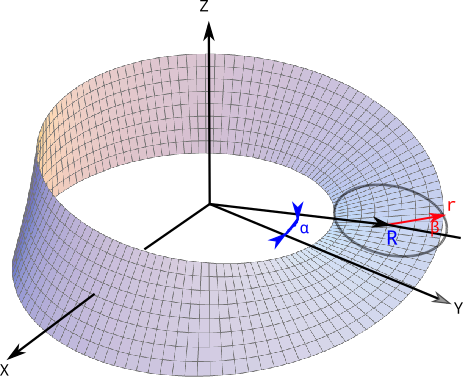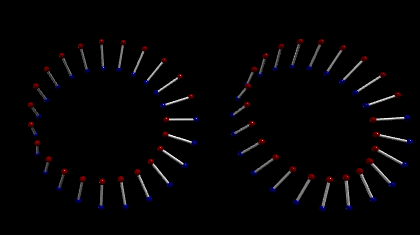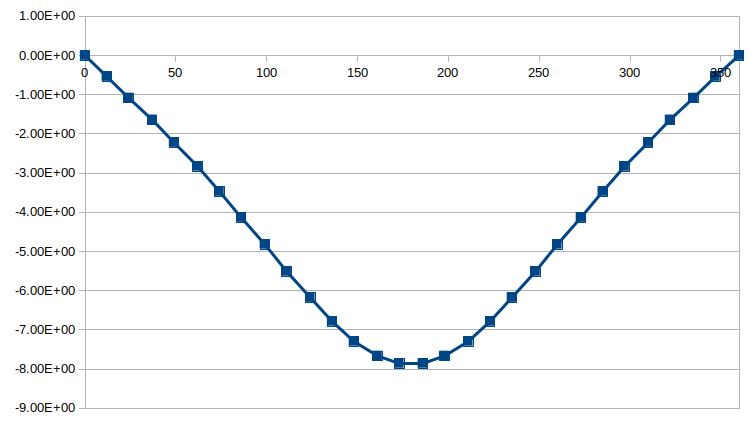## 1. 前言

NeoAtlantis应用科学和神秘学实验室根据一次神秘学研究，得知使用磁铁构成的莫比乌斯环可能具有 某些特殊的力学特性[*]。 根据提示，这种莫比乌斯环可以使用强力的永久磁铁构成，其中两个环分别安装在轮状的支撑结构上。 现在试图研究其中一个环对另一个环施加的力，尤其是对轮轴的力矩。

[*] 关于这一点，本文不会详细描述，如果您看完本文有何猜想，或者想进一步了解，请和作者联系

## 2. 研究方法

### 2.1 莫比乌斯环的数学模型$Z = R + r \textrm{sin}(\beta) = R + r \textrm{sin}(\frac{1}{2}\alpha)$

X 和 Y 的坐标，可以由 R 与 r在XY平面上的投影 两部分之和分解得到：

$X = [R + r \textrm{cos}(\beta)]\textrm{cos}(\alpha) = [R + r \textrm{cos}(\frac{1}{2}\alpha)]\textrm{cos}(\alpha)$ $Y = [R + r \textrm{cos}(\beta)]\textrm{sin}(\alpha) = [R + r \textrm{cos}(\frac{1}{2}\alpha)]\textrm{sin}(\alpha)$

### 2.2 磁铁相互作用的模型(上图：安培电流模型，下图：吉尔伯特磁荷模型）

$F = \frac{q_1q_2}{r^2}$

## 3 编程计算

### 3.1 安装了磁铁的莫比乌斯环

• 环的几何形状由上一章的公式指定
• 我们在一个圆周360度内，选取N=24个角度，将圆周均分，并根据公式得出莫比乌斯环的一个端点
• 在上述模型中，将 r 反向（180度），可以得到莫比乌斯环在每个截面上的另一个端点
• 这样的两个端点，分别是磁铁的N和S极，具有磁荷1和-1### 3.2 莫比乌斯环之间的相互作用力